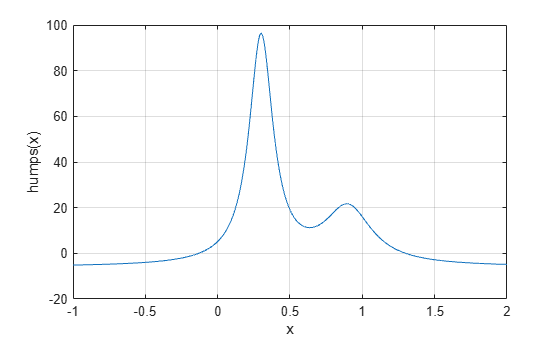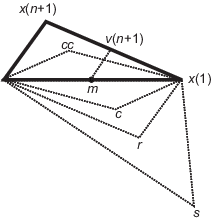Optimizing Nonlinear Functions

Minimizing Functions of One Variable

Given a mathematical function of a single variable, you can use the fminbnd function to find a local minimizer of the function in a given interval. For example, consider the humps.m function, which is provided with MATLAB®. The following figure shows the graph of humps.

x = -1:.01:2;
y = humps(x);
plot(x,y)
xlabel('x')
ylabel('humps(x)')
grid onTo find the minimum of the humps function in the range (0.3,1), use

x = fminbnd(@humps,0.3,1)
x = 0.6370

You can see details of the solution process by using optimset to create options with the Display option set to 'iter'. Pass the resulting options to fminbnd.

options = optimset('Display','iter');
x = fminbnd(@humps,0.3,1,options)

Func-count     x          f(x)         Procedure
1       0.567376      12.9098        initial
2       0.732624      13.7746        golden
3       0.465248      25.1714        golden
4       0.644416      11.2693        parabolic
5         0.6413      11.2583        parabolic
6       0.637618      11.2529        parabolic
7       0.636985      11.2528        parabolic
8       0.637019      11.2528        parabolic
9       0.637052      11.2528        parabolic

Optimization terminated:
the current x satisfies the termination criteria using OPTIONS.TolX of 1.000000e-04
x = 0.6370

The iterative display shows the current value of x and the function value at f(x) each time a function evaluation occurs. For fminbnd, one function evaluation corresponds to one iteration of the algorithm. The last column shows the procedure fminbnd uses at each iteration, a golden section search or a parabolic interpolation. For details, see Optimization Solver Iterative Display.

Minimizing Functions of Several Variables

The fminsearch function is similar to fminbnd except that it handles functions of many variables. Specify a starting vector x0 rather than a starting interval. fminsearch attempts to return a vector x that is a local minimizer of the mathematical function near this starting vector.

To try fminsearch, create a function three_var of three variables, x, y, and z.

function b = three_var(v)
x = v(1);
y = v(2);
z = v(3);
b = x.^2 + 2.5*sin(y) - z^2*x^2*y^2;

Now find a minimum for this function using x = -0.6, y = -1.2, and z = 0.135 as the starting values.

v = [-0.6,-1.2,0.135];
a = fminsearch(@three_var,v)

a =
0.0000   -1.5708    0.1803

Maximizing Functions

The fminbnd and fminsearch solvers attempt to minimize an objective function. If you have a maximization problem, that is, a problem of the form

$\underset{x}{\mathrm{max}}f\left(x\right),$

then define g(x) = –f(x), and minimize g.

For example, to find the maximum of tan(cos(x)) near x = 5, evaluate:

[x fval] = fminbnd(@(x)-tan(cos(x)),3,8)

x =
6.2832

fval =
-1.5574

The maximum is 1.5574 (the negative of the reported fval), and occurs at x = 6.2832. This answer is correct since, to five digits, the maximum is tan(1) = 1.5574, which occurs at x = 2π = 6.2832.

fminsearch Algorithm

fminsearch uses the Nelder-Mead simplex algorithm as described in Lagarias et al. . This algorithm uses a simplex of n + 1 points for n-dimensional vectors x. The algorithm first makes a simplex around the initial guess x0 by adding 5% of each component x0(i) to x0. The algorithm uses these n vectors as elements of the simplex in addition to x0. (The algorithm uses 0.00025 as component i if x0(i) = 0.) Then, the algorithm modifies the simplex repeatedly according to the following procedure.

Note

The keywords for the fminsearch iterative display appear in bold after the description of the step.

1. Let x(i) denote the list of points in the current simplex, i = 1,...,n + 1.

2. Order the points in the simplex from lowest function value f(x(1)) to highest f(x(n + 1)). At each step in the iteration, the algorithm discards the current worst point x(n + 1), and accepts another point into the simplex. [Or, in the case of step 7 below, it changes all n points with values above f(x(1))].

3. Generate the reflected point

 r = 2m – x(n + 1), (1)

where

 m = Σx(i)/n, i = 1...n, (2)

and calculate f(r).

4. If f(x(1)) ≤ f(r) < f(x(n)), accept r and terminate this iteration. Reflect

5. If f(r) < f(x(1)), calculate the expansion point s

 s = m + 2(m – x(n + 1)), (3)

and calculate f(s).

1. If f(s) < f(r), accept s and terminate the iteration. Expand

2. Otherwise, accept r and terminate the iteration. Reflect

6. If f(r) ≥ f(x(n)), perform a contraction between m and eitherx(n + 1) or r, depending on which has the lower objective function value.

1. If f(r) < f(x(n + 1)) (that is, r is better than x(n + 1)), calculate

 c = m + (r – m)/2 (4)

and calculate f(c). If f(c) < f(r), accept c and terminate the iteration. Contract outside

Otherwise, continue with Step 7 (Shrink).

2. If f(r) ≥ f(x(n + 1)), calculate

 cc = m + (x(n + 1) – m)/2 (5)

and calculate f(cc). If f(cc) < f(x(n + 1)), accept cc and terminate the iteration. Contract inside

Otherwise, continue with Step 7 (Shrink).

7. Calculate the n points

 v(i) = x(1) + (x(i) – x(1))/2 (6)

and calculate f(v(i)), i = 2,...,n + 1. The simplex at the next iteration is x(1), v(2),...,v(n + 1). Shrink

The following figure shows the points that fminsearch can calculate in the procedure, along with each possible new simplex. The original simplex has a bold outline. The iterations proceed until they meet a stopping criterion.Reference

 Lagarias, J. C., J. A. Reeds, M. H. Wright, and P. E. Wright. “Convergence Properties of the Nelder-Mead Simplex Method in Low Dimensions.” SIAM Journal of Optimization, Vol. 9, Number 1, 1998, pp. 112–147.